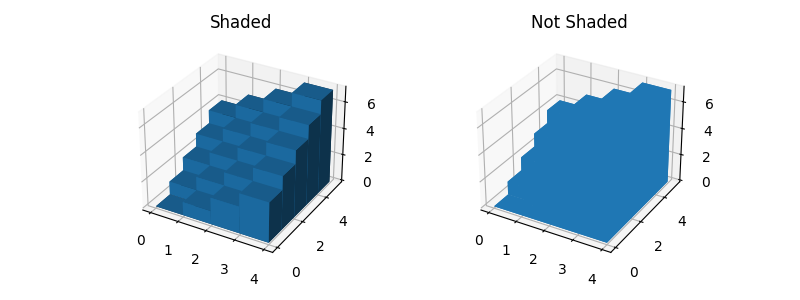# Demo of 3D bar charts#

A basic demo of how to plot 3D bars with and without shading.import numpy as np
import matplotlib.pyplot as plt

# setup the figure and axes
fig = plt.figure(figsize=(8, 3))

# fake data
_x = np.arange(4)
_y = np.arange(5)
_xx, _yy = np.meshgrid(_x, _y)
x, y = _xx.ravel(), _yy.ravel()

top = x + y
bottom = np.zeros_like(top)
width = depth = 1

ax1.bar3d(x, y, bottom, width, depth, top, shade=True)